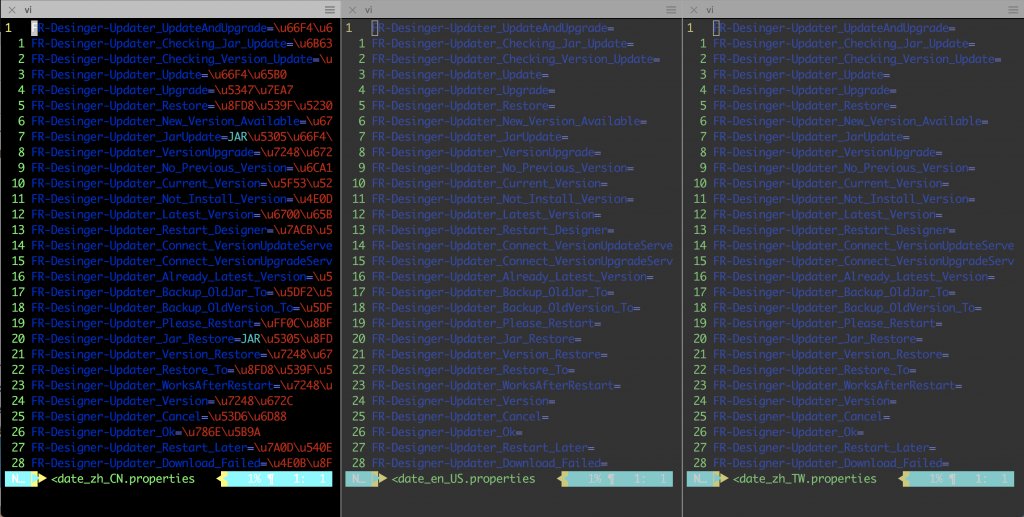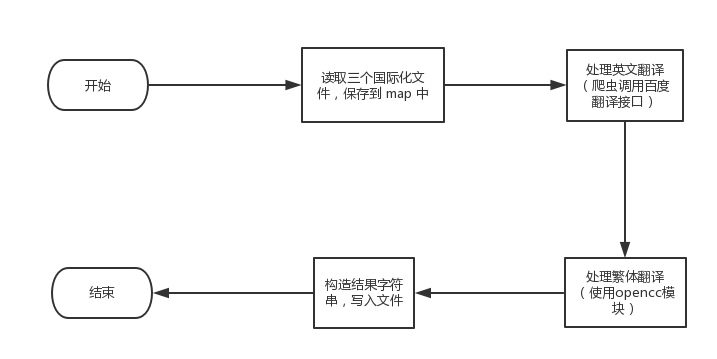2018年8月21日

# 入门 PYTHON 脚本编程

—— 以处理国际化问题为例

• 介绍 Python 语言
• 用自动化脚本辅助日常工作的思想
• 读完之后，可快速上手，编写简单的脚本程序
• 为以后学习“机器学习”等 Python 擅长的领域做铺垫

## 一、抛出应用问题## 二、Python 基础

#### 写在前面

• Python 是一种解释型、面向对象、动态数据类型的高级程序设计语言。
• Python 开源、跨平台，社区活跃，类库非常多。
• Python 易于入门，功能强大。常被比作“胶水”和“万金油”。
• Python 分 Python2 和 Python3 两个版本，差别不大，本文基于 Python3。
• “人生苦短，我用 Python！”

#### 运行一个 HelloWorld

`print("Hello World")`

（演示）

#### 基本语法

• 解释型语言，只能在运行时发现错误
• 有一个交互式 shell（类似 node）
• 包管理器：pip
• 变量是动态类型
• 每行一句话，不建议使用分号
• 单引号、双引号、三引号
• 用缩进表示语句块
• 与或非：and、or、not

#### 常用数据结构

1) list（列表），常用操作：切片

```In : l = [1, 2, 3, 4, 5]

In : l[1:3]
Out: [2, 3]```

2) tuple（元组，不可变列表）

3) map（字典）

4) set（集合），常用操作：去重

```In : l = [1, 1, 1, 2, 2, 3]

In : set(l)
Out: {1, 2, 3}```

#### 最常见语法糖

1) 多变量赋值

```In : a, b, c = 1, 2, "john"

In : a
Out: 1

In : b
Out: 2

In : c
Out: 'john'```

2) With 语句（简化异常处理）

```In : with open('update.properties') as f:

3) 列表推导式（轻量级循环）

```In : l2 = [n for n in l if n > 1]

In : l2
Out: [2, 2, 3]```

#### 模块机制

```In : import os

In : os.listdir()
Out:
['update_en_US.properties',
'update_zh_TW.properties',
'update.properties',
'update_zh_CN.properties',
'.git']```

## 三、国际化脚本程序演示## 四、国际化脚本程序剖析

• 模块使用
• 文件IO
• 文本处理
• 简单爬虫

#### 主结构

```#!/usr/bin/env python3
# encoding: utf-8
import os
import collections
import ipdb
import codecs
import opencc
import translater

def main():
base = os.getcwd()
locale_CN = None
locale_TW = None
locale_EN = None
for file in os.listdir(base):
if file.endswith('zh_CN.properties'):
locale_CN = file
continue
if file.endswith('zh_TW.properties'):
locale_TW = file
continue
if file.endswith('en_US.properties'):
locale_EN = file
continue
if not locale_CN or not locale_TW or not locale_EN:
print('没有找到对应的国际化文件，请检查！')
return

map_CN = get_kvmap_in_locale_file(locale_CN)

process_TW(map_CN, locale_TW)
process_EN(map_CN, locale_EN)
```

#### 0) 用到的模块

• os，与操作系统有关的功能
• collections，提供一些特殊的集合
• codecs，编码转换
• opencc，台湾繁体翻译
• requests，发起网络请求

#### 1) 将国际化文件读取为 map

```def get_kvmap_in_locale_file(file):
kvmap = collections.OrderedDict()
with open(file) as f:
contentList = content.splitlines()
for line in contentList:
# ipdb.set_trace()
if not line or line.startswith('#'):
continue
if '=' not in line:
print('!!!\n', line)
k, v = line.split('=', 1)
k = k.strip()
kvmap[k] = v
return kvmap```

#### 2) 爬虫调用百度翻译接口进行英文翻译（封装为 translater 模块）

```#!/usr/bin/env python3
import requests

# 中译英
def zh2en(content):
data = {
'from': 'zh',
'to': 'en',
'query':content,
}
return _translate(data)

# 英译中
def en2zh(content):
data = {
'from': 'en',
'to': 'zh',
'query':content,
}
return _translate(data)

def _translate(data):
# 手机版api
url = 'http://fanyi.baidu.com/basetrans'
headers = {"User-Agent":"Mozilla/5.0 (Linux; Android 5.1.1; Nexus 6 Build/LYZ28E) AppleWebKit/537.36 (KHTML, like Gecko) Chrome/63.0.3239.84 Mobile Safari/537.36"}
result = response.json()['trans']['dst']
return result

if __name__=="__main__":
print(zh2en('你好，世界'))
print(en2zh('Hello, world'))```

#### 3) 处理英文翻译

```def process_EN(map_CN, locale_EN):
# 处理英文翻译
map_EN = get_kvmap_in_locale_file(locale_EN)
error_keys = []
result_EN = ''
for k, v in map_EN.items():
if not v and map_CN[k]:
try:
v_CN = codecs.decode(map_CN[k], 'unicode_escape')
v = translater.zh2en(v_CN)
print(k, v_CN, v)
except Exception as e:
print(str(e))
error_keys.append(k)
result_EN += k + '=' + v + '\n'
with open(locale_EN, 'w') as f:
f.write(result_EN)
print('请手动处理以下英文key: ')
for k in error_keys:
print(k)```

#### 4) 处理台湾翻译

```def process_TW(map_CN, locale_TW):
# 处理台湾翻译
map_TW = get_kvmap_in_locale_file(locale_TW)
error_keys = []
result_TW = ''
for k, v in map_TW.items():
if not v and map_CN[k]:
try:
v_CN = codecs.decode(map_CN[k], 'unicode_escape')
v_TW = opencc.convert(v_CN, config='s2twp.json')
v_TW = codecs.encode(v_TW, 'unicode_escape').decode()
v = v_TW.upper().replace('\\U', '\\u')
except Exception as e:
print(str(e))
error_keys.append(k)
result_TW += k + '=' + v + '\n'
with open(locale_TW, 'w') as f:
f.write(result_TW)
print('请手动处理以下key: ')
for k in error_keys:
print(k)```

`alias addtrans='/Users/plough/codes/增加翻译/addtrans.py'`

## 六、答疑

#### 1) 为什么使用 OpenCC，而不是百度翻译？

1. 百度翻译是直译，台湾繁体除了字体改变，某些词汇也有变化。OpenCC 的转换效果更好。（现在的百度翻译已经变得更智能了）
2. 演示第三方类库的使用

#### 2) Python 的用途？

Python 是“万金油”，至少有以下应用：

• 自动化脚本；
• web 后台；
• 科学计算；
• 数据挖掘；
• 机器学习；
• 图像处理；
• 游戏编程；
• 爬虫；
• GUI 编程；
• 嵌入式编程

Python 是“胶水”，可以把其他语言编写的程序串联起来。所以有无限的扩展性。

#### 3) Python 效率低下？

• 第一次运行时，会编译出 .pyc 文件，再次运行速度会变快；
• 如果有必要，关键部分，可以用 C 语言扩展。

#### 4) 脚本常见用途？

• 处理国际化问题
• 找出项目源码 js 里多余的逗号
• 文本处理
• 系统管理
• 自动化运维、自动化测试
• 定时任务
• 爬取数据
• 抢月饼